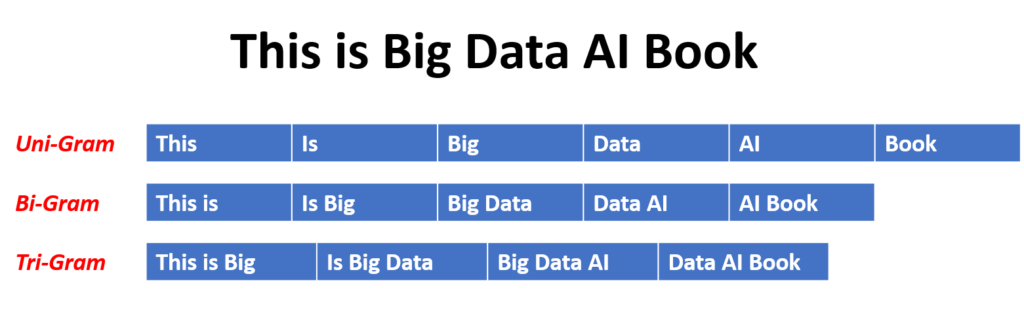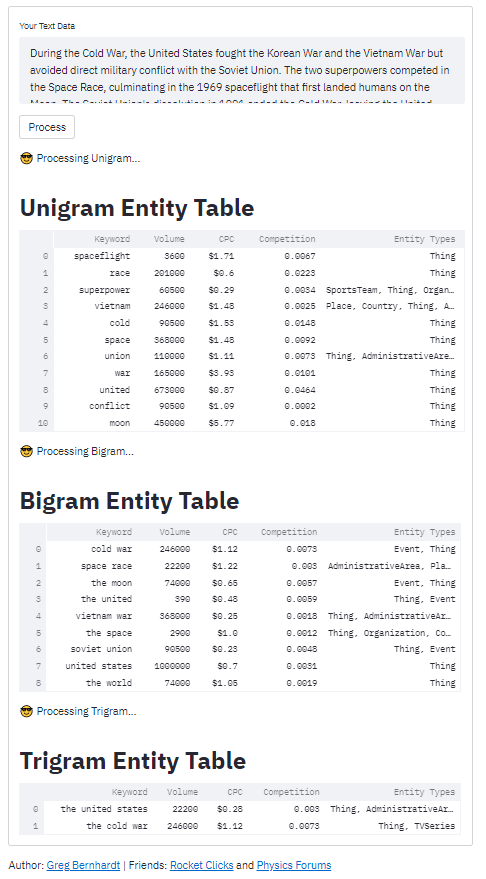Common Topics: data, list, api, entities, text

The days where content SEO was simply copywriting are over. Modern content SEO now employs massive resources for technical analysis for the words you write/manage. Actually, this has been the case for nearly 10 years now with the introduction of machine learning in search engines. The tools are now widely available to SEOs to achieve a more granular and sophisticated understanding of the content they write, rather from just a subjective perspective.

When you are able to break down the elements of your written content, you are able to extract insights in the form of patterns, relationships, and granular search metrics that better inform you as an SEO as to the meaning of your content. The better we understand the meaning of our content and the signals we build within that content, the more successful our creations and predictions for those creations will be.

In this tutorial I’ll break down my app script that analyzes a body of text for n-grams, entities, and search metrics, so you can build off it for your own purposes.

As defined by Wikipedia: “In the fields of computational linguistics and probability, an n-gram is a contiguous sequence of n items from a given sample of text or speech.”

The site Devopedia has this handy graphic example of how text is broken into n-grams.Now let’s find out how to build a framework to help break down your content into n-grams and start some basic analysis.

Not interested in the tutorial? Head straight for the app here.

## Import and Install Modules

• nltk: NLP module handles the n-graming and word type tagging
• pandas: for storing and displaying the results
• requests: for making API calls to Keyword Sufer API
• json: for processing the Keyword Surfer API response

First, let’s install the nltk module which you won’t like have already.  If you are using Google Colab put an exclamation mark at the beginning.

`pip3 install nltk`

Now let’s import all the modules as described above, into the script.

```import nltk
from nltk.util import ngrams
import requests
import json
import pandas as pd```

## Build N-Grams from Provided Text

We’re going to start off with a few functions. I decided to use functions because my app will process 1, 2, 3-gram tables when this script as is, will only process one of them of your choosing. You can automate at the end for all 3 by using a simple while loop. This first function breaks the text into what n-gram you choose later on.

1.  Send the text data into the nltk ngram function that returns a list of those n-grams. It takes two parameters. The first is a tokenized version of that text which it processes in the word_tokenize function first and the second parameter is whatever whole number n-gram you want.
2.  We join all the n-grams that are returned into a single list
3.  For Unigrams we want to tag each tokenized word and filter out anything that isn’t a noun or action verb (present participle)
4.  Return the list back to the main script
```def extract_ngrams(data, num):
n_grams = ngrams(nltk.word_tokenize(data), num)
gram_list = [ ' '.join(grams) for grams in n_grams]

if num == 1:
query_tokens = nltk.pos_tag(gram_list)
query_tokens = [x for x in query_tokens if x in ['NN','NNS','NNP','NNPS','VBG','VBN']]
query_tokens = [x for x in query_tokens]

return query_tokens```

## Detect Entities from N-Grams

Next, we tackle the function that will take the list of n-grams and pass it to the Google Knowledge Graph (GKG) API to check if it’s an entity.

1.  Create a variable to store the n-grams that end up as entities and a variable for your GKG API key.
2.  Loop through all the n-grams in the list
3.  Make a request to GKG using n-gram, API key, and limiting to first result found (highest score, most likely)
4.  Load API JSON response into a JSON object to parse
5.  Parse JSON object for “type”, aka entity category, and resultScore, aka their relevancy confidence metric. Some requests are not entities and will break the script so we use a Try/Except method. If the Except is triggered, we make a score of 0 and a label of none.
6.  In the JSON data, the entity labels are in a list because entities often have more than one label or category. We loop over this list and create a string delineated list. In the end, we remove the last hanging comma and create a space between the comma and the next label.
7.  If a label doesn’t equal none and has a resultScore of greater than 500 let’s build a list for that n-gram that stores the n-gram, its score, and its labels. Then we append that list to a master list to store all the entity data. So we’ll have a list of lists. We then return that list of lists back to the main script.
```def kg(keywords):

kg_entities = []
apkikey=''

for x in keywords:

try:
getlabel = data['itemListElement']['result']['@type']
score = round(float(data['itemListElement']['resultScore']))
except:
score = 0
getlabel = ['none']

labels = ""

for item in getlabel:
labels += item + ","
labels = labels[:-1].replace(",",", ")

if labels != ['none'] and score > 500:
kg_subset = []
kg_subset.append(x)
kg_subset.append(score)
kg_subset.append(labels)

kg_entities.append(kg_subset)
return kg_entities```

## Gather Entity Search Metrics

For our final function, we build our API call to Keyword Surfer to get volume, CPC, and competition score. Then we build the dataframe with all of our information.

1.  Create a list with only the entity types.
2.  Create a list with only the entity names.
3.  Convert the entity names list to a string so we can feed it to the URL API.
4.  Build the Keyword Surfer API call using the keyword string-list. Please do not abuse this API or we may find it blocked at some point.
5.  Return the JSON response to seo_data variable.
6.  Build the empty dataframe which we’ll use to store the data in later.
7.  Loop through the entities we fed to the API.
8.  Some entities won’t have data. We detect and assign a value manually for CPC and competition.
9.  We build a dictionary object using the data for each entity and then append it to the dataframe we created earlier.
10.  We format the dataframe with alignment and sorting by Volume and then return the dataframe to the main script.
```def surfer(entities,gram):
entities_type = [x for x in entities]
entities = [x for x in entities]
keywords = json.dumps(entities)

url2 = 'https://db2.keywordsur.fr/keyword_surfer_keywords?country=us&keywords=' + keywords
response2 = requests.get(url2,verify=True)

d = {'Keyword': [], 'Volume': [], 'CPC':[], 'Competition':[], 'Entity Types':[]}
df = pd.DataFrame(data=d)
counter=0

for x in seo_data:

if seo_data[x]["cpc"] == '':
seo_data[x]["cpc"] = 0.0

if seo_data[x]["competition"] == '':
seo_data[x]["competition"] = 0.0

new = {"Keyword":word,"Volume":str(seo_data[x]["search_volume"]),"CPC":"\$"+str(round(float(seo_data[x]["cpc"]),2)),"Competition":str(round(float(seo_data[x]["competition"]),4)),'Entity Types':entities_type[counter]}
df = df.append(new, ignore_index=True)
counter +=1

df.style.set_properties(**{'text-align': 'left'})
df.sort_values(by=['Volume'], ascending=True)
return df```

## Provide Text and Call Functions

We arrive now at the actual start of the script where the data variable should contain the text you want to analyze.  We clean the text a little to be free of some pesky punctuation that sometimes breaks analysis. Certainly, there is a more efficient way of handling it, but this works fine for now. Feel free to add more replacements.

```data = ''
data = data.replace('\\','')
data = data.replace(',','')
data = data.replace('.','')
data = data.replace(';','')```

Here we send the data from above to the first function we created that processes for ngrams. The second parameter number is the number of ngram you want to process.

• 1 = unigram
• 2 = bigram
• 3 = trigram …

Then we send the ngram lists to the Google Knowledge Graph API for entity detection. Lastly, we send the entities to the Keyword Surfer API for metrics, and finally, we display the dataframe.

```keywords = extract_ngrams(data, 3)
entities = kg(keywords)
df = surfer(entities,2)
df```

All that is left is to save the dataframe to file as a CSV.

`pd.to_csv(df)`

## Conclusion

Here is the output as provided by the corresponding app. Note my app loops through the first 3 ngrams and has plenty of streamlit app formatting added. You’ll need to build a simple while loop to achieve this. Click the image to visit the app.Now you have the framework to begin analyzing your content at a more surgical level. Remember to try and make my code even more efficient.  Now get out there and try it out! Follow me on Twitter and let me know your applications and ideas!